#### 期刊菜单

A BLDC Design Suitable for Electro-Mechanical Braking Device
DOI: 10.12677/DSC.2019.82011, PDF, HTML, XML, 下载: 843  浏览: 1,741

Abstract: For a new type of rail transit brake device, a drive motor suitable for such a device is designed. The motors designed in this paper are generally used in automatic control equipment. But this motor is different from a normal BLDC and operates normally in a stalled state. This paper introduces the composition and working principle of BLDC, deeply analyzes the selection of rotor magnetic circuit and winding form, permanent magnet material, and adopts the design analysis method of equivalent magnetic circuit, and uses RMxprt to calculate the BLDC. Finally, the optimal design is determined. The designed motor meets the requirements of the device and has good electromagnetic performance.

1. 引言

2. 基本组成以及工作原理

2.1. 基本组成Figure 1. Structure of BLDC

2.2. 工作原理

3. 列车电机械制动装置驱动电机关键性能指标

3.1. 电机械制动驱动电机设计目标参数Table 1. Domestic common urban rail unit brake maximum brake shoe pressure

3.2. 连续输出堵转转矩

${T}_{e}=\frac{21\text{\hspace{0.17em}}\text{KN}}{1.6\text{\hspace{0.17em}}\text{KN}/\text{Nm}×85%}=15.4\text{\hspace{0.17em}}\text{Nm}$

3.3. 空载转速

$\frac{{n}_{\mathrm{max}}}{L}=300\text{\hspace{0.17em}}\text{r}/\text{min}$4. 电磁设计

4.1. 转子磁路设计

4.2. 永磁体的选用

4.3. 极弧系数

${\alpha }_{p}=\frac{{b}_{p}}{{\tau }_{1}}$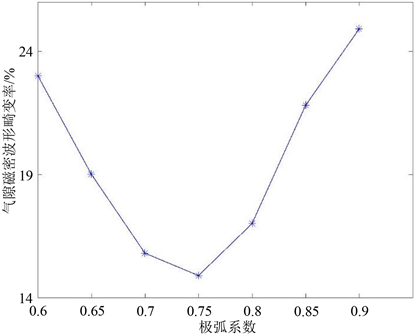Figure 2. Air gap magnetic distortion waveform distortion rate under different pole arc coefficients

4.4. 气隙长度的选择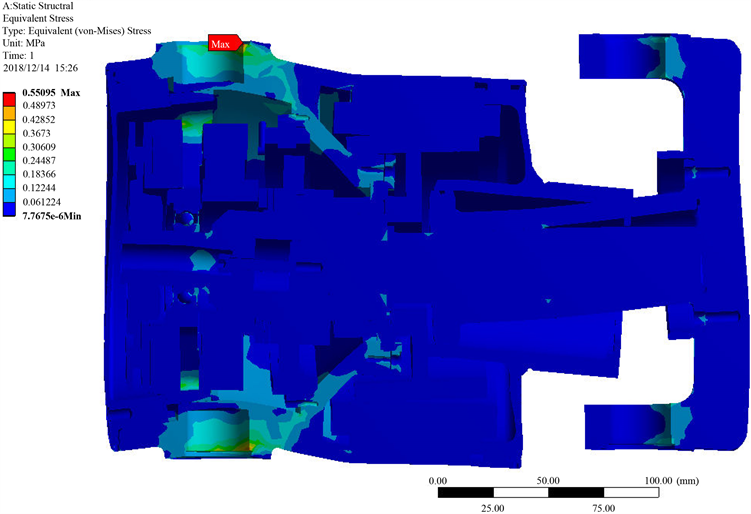Figure 3. Equivalent stress distribution cloudTable 4. Brake cylinder deformation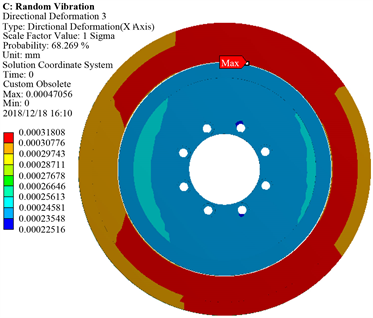Figure 4. Vertical deformation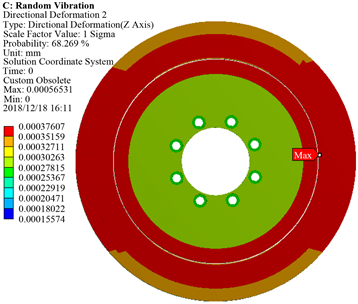Figure 5. Longitudinal deformation

4.5. 极槽配合

4.6. 绕组设计

AAZBXXCYAAZBXXCYAAZBXCCYAZZBXCCYAZZBXCCYAZBBXCYYAZBBXCYYAZBBXCY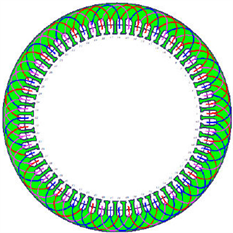Figure 6. Armature winding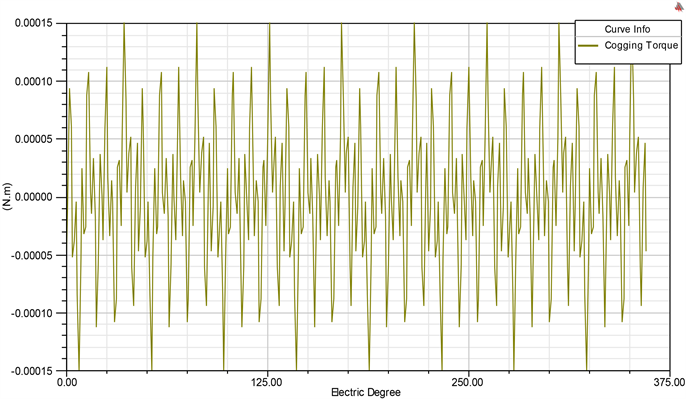Figure 7. Cogging torque in two teeth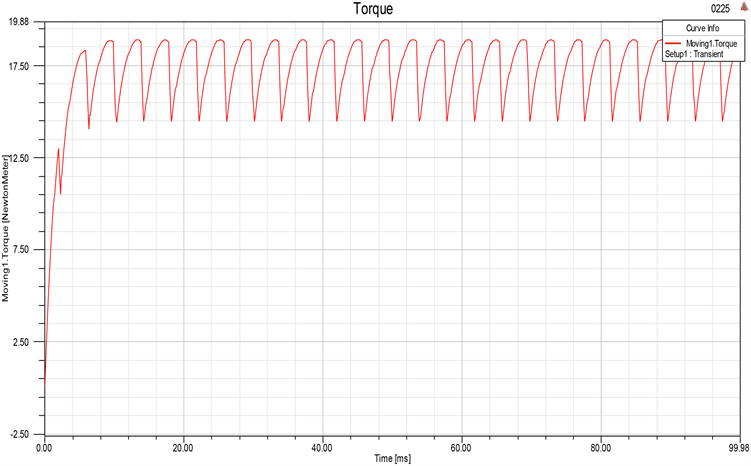Figure 8. Te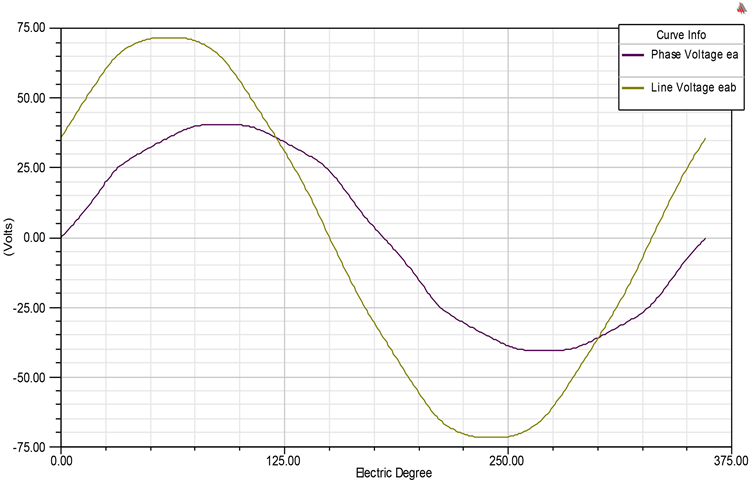Figure 9. Induced winding voltages at rated speedFigure 10. Air-gap flux densityTable 6. Electromagnetic performance parameters of brushless DC torque motor for electromechanical

5. 电机械用无刷直流力矩电机电磁参数计算

6. 结语

  Pan, S.H., Song, Z.D. and Wang, X.P. (2016) Electro-Mechanical Braking Force Distribution Strategy for Electric Vehicle. IEEE International Conference on Communication Problem-Solving, Guilin, 16-18 October 2015, 387-390.  朱剑波. 无刷直流电机控制系统的仿真与分析[J]. 国外电子测量技术, 2013, 32(12): 25-30.  夏长亮, 方红伟. 永磁无刷直流电机及其控制[J]. 电工技术学报, 2012(3): 25-34.  王艳萍. 航海用无刷直流力矩电动机的设计研究及Ansoft仿真[D]: [硕士学位论文]. 西安: 西安电子科技大学, 2010.  唐任远. 现代永磁电机理论与设计[M]. 北京: 机械工业出版社, 2011.  李运洲. 基于Modelica的无刷直流电机设计与仿真[D]: [硕士学位论文]. 武汉: 华中科技大学, 2017.